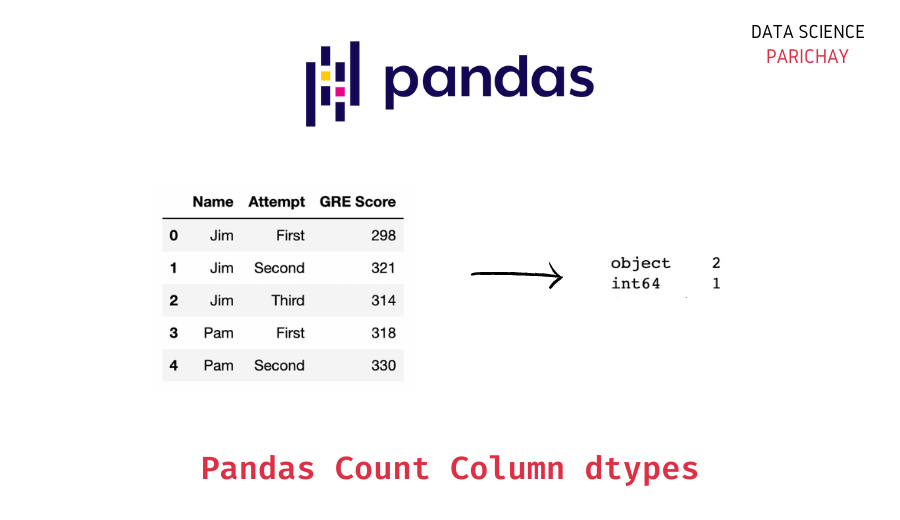# Get Count of dtypes in a Pandas DataFrame

In this tutorial, we will look at how to get a count of the dtypes (data types of columns) in a pandas dataframe with the help of some examples.

## How to get the datatype for each column in a Pandas Dataframe?

You can use the pandas dataframe `dtypes` attribute to get the data type of each column in a dataframe. The following is the syntax –

`df.dtypes`

Let’s look at an example.

```import pandas as pd

df = data['frame']
# dtype of each column
print(df.dtypes)```

Output:

```alcohol                         float64
malic_acid                      float64
ash                             float64
alcalinity_of_ash               float64
magnesium                       float64
total_phenols                   float64
flavanoids                      float64
nonflavanoid_phenols            float64
proanthocyanins                 float64
color_intensity                 float64
hue                             float64
od280/od315_of_diluted_wines    float64
proline                         float64
target                            int64
dtype: object```

Here we load the wine dataset using `sklearn.datasets` and then proceed to see the dtypes of all the columns in the dataframe using the dataframe’s `dtypes` attribute.

Alternatively, you can also use the dataframe `info()` function to see the data type of columns in a dataframe.

```# dataframe info
print(df.info())```

Output:

```<class 'pandas.core.frame.DataFrame'>
RangeIndex: 178 entries, 0 to 177
Data columns (total 14 columns):
#   Column                        Non-Null Count  Dtype
---  ------                        --------------  -----
0   alcohol                       178 non-null    float64
1   malic_acid                    178 non-null    float64
2   ash                           178 non-null    float64
3   alcalinity_of_ash             178 non-null    float64
4   magnesium                     178 non-null    float64
5   total_phenols                 178 non-null    float64
6   flavanoids                    178 non-null    float64
7   nonflavanoid_phenols          178 non-null    float64
8   proanthocyanins               178 non-null    float64
9   color_intensity               178 non-null    float64
10  hue                           178 non-null    float64
11  od280/od315_of_diluted_wines  178 non-null    float64
12  proline                       178 non-null    float64
13  target                        178 non-null    int64
dtypes: float64(13), int64(1)
memory usage: 19.6 KB
None```

We get the column names and their respective dtypes along with other information about the dataframe.

📚 Data Science Programs By Skill Level

Introductory

Intermediate ⭐⭐⭐

🔎 Find Data Science Programs 👨‍💻 111,889 already enrolled

Disclaimer: Data Science Parichay is reader supported. When you purchase a course through a link on this site, we may earn a small commission at no additional cost to you. Earned commissions help support this website and its team of writers.

## Count of all dtypes in Pandas Dataframe

You can use the pandas `DataFrame.dtypes.value_counts()` function to get a count of each dtype in the dataframe. Here, we apply the pandas `value_counts()` function to the pandas series returned by the `dtypes` attribute. The following is the syntax –

```# count of each dtype in dataframe
df.dtypes.value_counts()```

Let’s look at an example. Let’s apply the above method to the wine dataset that we have loaded.

```# count of each dtype in the wine dataset
df.dtypes.value_counts()```

Output:

```float64    13
int64       1
dtype: int64```

We get the count of different column data types present in the dataframe. You can see that there are 13 columns with the type `float64` and one column with the type `int64`.

Let’s look at another example. This time, we create a dataframe from scratch.

```import pandas as pd

# create a dataframe of GRE scores of two students
df = pd.DataFrame({
'Name': ['Jim', 'Jim', 'Jim', 'Pam', 'Pam'],
'Attempt': ['First', 'Second', 'Third', 'First', 'Second'],
'GRE Score': [298, 321, 314, 318, 330]
})
# display the dataframe dtypes
print(df.dtypes)
print("--------")
# display the dtypes counts
print(df.dtypes.value_counts())```

Output:

```Name         object
Attempt      object
GRE Score     int64
dtype: object
--------
object    2
int64     1
dtype: int64```

Here we create a dataframe of GRE scores of two students. You can see that the “Name” and the “Attempt” columns are of `object` type while the “GRE Score” column is of `int64` type.

You might also be interested in –

•